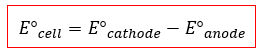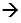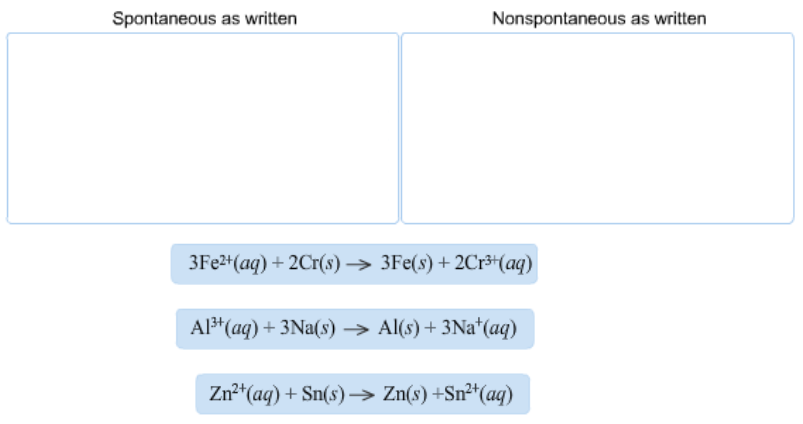# Problem: Based on the sign of E°cell, classify these reactions as spontaneous or nonspontaneous as written. Assume standard conditions.

###### FREE Expert Solution

We´re asked to classify these reactions based on the sign of E°cell assuming standard conditions.

Remember that cell > 0 is spontaneous, while cell < 0 is nonspontaneous.

To determine that, we require to calculate E°cell using the following equation:When a balanced reaction is given, identify the anode and the cathode half-reactions by determining the changes in oxidation states of each species based on the given reaction:

Recall:

Cathodereductionoxidation number decreases

Anodeoxidationoxidation number increases

93% (198 ratings)###### Problem Details

Based on the sign of E°cell, classify these reactions as spontaneous or nonspontaneous as written. Assume standard conditions.